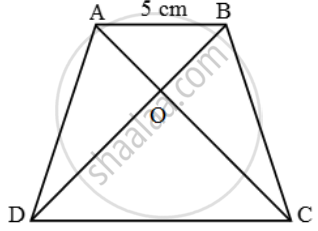# In figure, AO/OC=BO/OD=1/2 and AB = 5 cm. Find the value of DC. - Mathematics

Sum

In figure, \frac{AO}{OC}=\frac{BO}{OD}=\frac{1}{2} and AB = 5 cm. Find the value of DC.

#### Solution

In ∆AOB and ∆COD, we have

∆AOB = ∆COD [Vertically opposite angles]

\frac{AO}{OC}=\frac{BO}{OD}So, by SAS-criterion of similarity, we have

∆AOB ~ ∆COD

\Rightarrow \frac{AO}{OC}=\frac{BO}{OD}=\frac{AB}{DC}

\Rightarrow \frac{1}{2}=\frac{5}{DC} [∵ AB = 5 cm]

⇒ DC = 10 cm

Concept: Similarity
Is there an error in this question or solution?